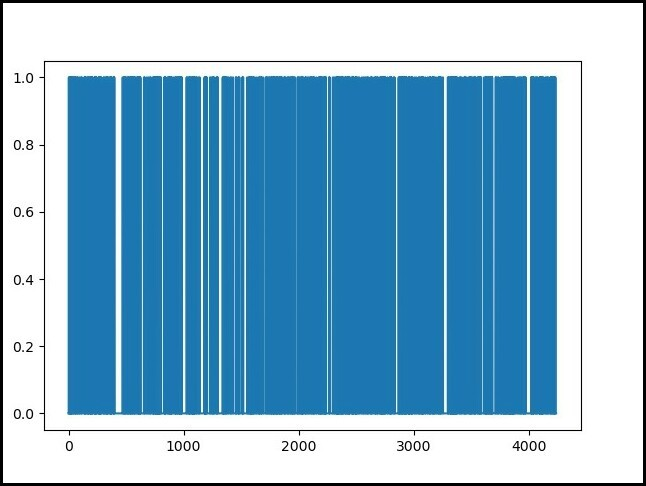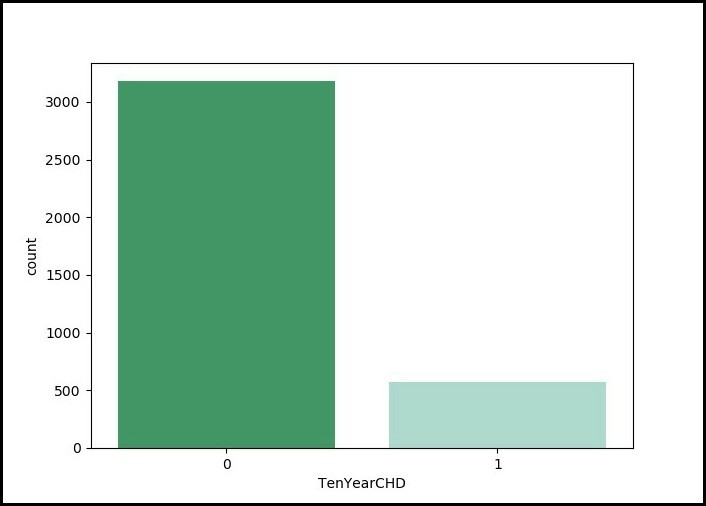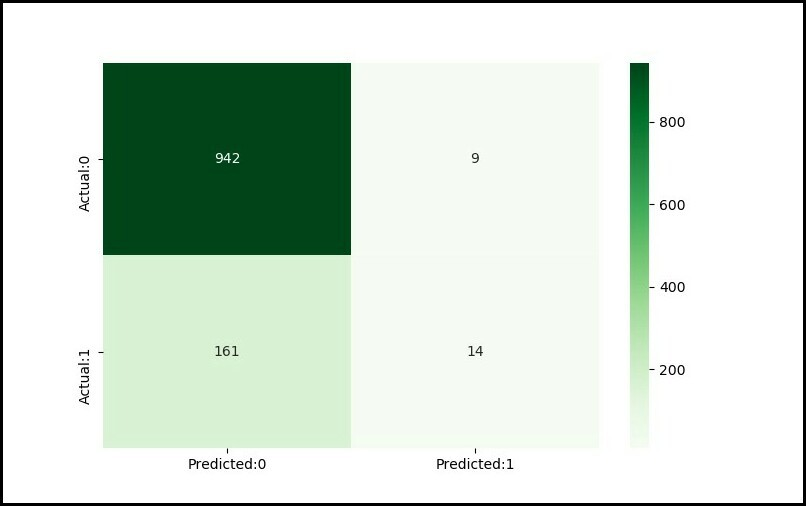# ML | Heart Disease Prediction Using Logistic Regression .

World Health Organization has estimated that four out of five cardiovascular diseases(CVD) deaths are due to heart attacks. This whole research intends to pinpoint the ratio of patients who possess a good chance of being affected by CVD and also to predict the overall risk using Logistic Regression.

What is Logistic Regression ?
Logistic Regression is a statistical and machine-learning techniques classifying records of a dataset based on the values of the input fields . It predicts a dependent variable based on one or more set of independent variables to predict outcomes . It can be used both for binary classification and multi-class classification. To know more about it, click here .

 `import` `pandas as pd ` `import` `pylab as pl ` `import` `numpy as np ` `import` `scipy.optimize as opt ` `import` `statsmodels.api as sm ` `from` `sklearn ``import` `preprocessing ` `'exec(% matplotlib inline)'` `import` `matplotlib.pyplot as plt ` `import` `matplotlib.mlab as mlab ` `import` `seaborn as sn `

Data Preparation :
The dataset is publically available on the Kaggle website, and it is from an ongoing cardiovascular study on residents of the town of Framingham, Massachusetts. The classification goal is to predict whether the patient has 10-years risk of future coronary heart disease (CHD). The dataset provides the patients’ information. It includes over 4,000 records and 15 attributes.

 `# dataset ` `disease_df ``=` `pd.read_csv(``"../input / framingham.csv"``) ` `disease_df.drop([``'education'``], inplace ``=` `True``, axis ``=` `1``) ` `disease_df.rename(columns ``=``{``'male'``:``'Sex_male'``}, inplace ``=` `True``) ` ` `  `# removing NaN / NULL values ` `disease_df.dropna(axis ``=` `0``, inplace ``=` `True``) ` `print``(disease_df.head(), disease_df.shape) ` `print``(disease_df.TenYearCHD.value_counts()) `

Output :

```
Sex_male  age  currentSmoker  ...  heartRate  glucose  TenYearCHD
0         1   39              0  ...       80.0     77.0           0
1         0   46              0  ...       95.0     76.0           0
2         1   48              1  ...       75.0     70.0           0
3         0   61              1  ...       65.0    103.0           1
4         0   46              1  ...       85.0     85.0           0

[5 rows x 15 columns] (3751, 15)
0    3179
1     572
Name: TenYearCHD, dtype: int64
```

Code: Ten Year’s CHD Record of all the patients available in the dataset :

 `# counting no. of patients affected with CHD ` `plt.figure(figsize ``=` `(``7``, ``5``)) ` `sn.countplot(x ``=``'TenYearCHD'``, data ``=` `disease_df,  ` `             ``palette ``=``"BuGn_r"` `) ` `plt.show() `

Output : Graph Display :Code: Counting number of patients affected by CHD where (0= Not Affected ; 1= Affected) :

 `laste ``=` `disease_df[``'TenYearCHD'``].plot() ` `plt.show(laste) `

Output: Graph Display :Code : Training and Test Sets: Splitting Data | Normalization of the Dataset

 `X ``=` `np.asarray(disease_df[[``'age'``, ``'Sex_male'``, ``'cigsPerDay'``,  ` `                           ``'totChol'``, ``'sysBP'``, ``'glucose'``]]) ` `y ``=` `np.asarray(disease_df[``'TenYearCHD'``]) ` ` `  `# normalization of the datset ` `X ``=` `preprocessing.StandardScaler().fit(X).transform(X) ` ` `  `# Train-and-Test -Split ` `from` `sklearn.model_selection ``import` `train_test_split ` `X_train, X_test, y_train, y_test ``=` `train_test_split(  ` `        ``X, y, test_size ``=` `0.3``, random_state ``=` `4``) ` `print` `(``'Train set:'``, X_train.shape,  y_train.shape) ` `print` `(``'Test set:'``, X_test.shape,  y_test.shape) `

Output :

```Train Set :
(2625, 6) (2625, )

Test Set :
(1126, 6) (1126, )
```

Code: Modeling of the Dataset | Evaluation and Accuracy :

 `from` `sklearn.linear_model ``import` `LogisticRegression ` `logreg ``=` `LogisticRegression() ` `logreg.fit(X_train, y_train) ` `y_pred ``=` `logreg.predict(X_test) ` ` `  `# Evaluation and accuracy ` `from` `sklearn.metrics ``import` `jaccard_similarity_score ` `print``('') ` `print``(``'Accuracy of the model in jaccard similarity score is = '``,  ` `      ``jaccard_similarity_score(y_test, y_pred)) `

Output :

```Accuracy of the model in jaccard similarity score is = 0.8490230905861457
```

Code: Using Confusion Matrix to find the Acuuracy of the model :

 `# Confusion matrix  ` `from` `sklearn.metrics ``import` `confusion_matrix, classification_report ` ` `  `cm ``=` `confusion_matrix(y_test, y_pred) ` `conf_matrix ``=` `pd.DataFrame(data ``=` `cm,  ` `                           ``columns ``=` `[``'Predicted:0'``, ``'Predicted:1'``],  ` `                           ``index ``=``[``'Actual:0'``, ``'Actual:1'``]) ` `plt.figure(figsize ``=` `(``8``, ``5``)) ` `sn.heatmap(conf_matrix, annot ``=` `True``, fmt ``=` `'d'``, cmap ``=` `"Greens"``) ` `plt.show() ` ` `  `print``(``'The details for confusion matrix is ='``) ` `print` `(classification_report(y_test, y_pred)) ` ` `  `# This code is contributed by parna_28 . `

Output :

```
The details for confusion matrix is =
precision    recall  f1-score   support

0       0.85      0.99      0.92       951
1       0.61      0.08      0.14       175

accuracy                           0.85      1126
macro avg       0.73      0.54      0.53      1126
weighted avg       0.82      0.85      0.80      1126
```

Confusion Matrix :My Personal Notes arrow_drop_upCheck out this Author's contributed articles.

If you like GeeksforGeeks and would like to contribute, you can also write an article using contribute.geeksforgeeks.org or mail your article to contribute@geeksforgeeks.org. See your article appearing on the GeeksforGeeks main page and help other Geeks.

Please Improve this article if you find anything incorrect by clicking on the "Improve Article" button below.